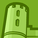Announcements
Product Release Webinar: Qlik Insider airing December 6! REGISTER TODAY!
cancel
Showing results for
Did you mean:Creator II

## Set analysis to equal a calculated concatenated value

Hello All!

This might sound simple, but I'm struggling with it... I'm trying to write a set analysis statement that does a calculation if a combined value equals another. So, for example

I have some fields to get prices:

 Area Price ABC1 1 ABC2 2 ABC3 3

I also have another set of information that has the area, but the leading number changes based on a calculation to work out the quantity.

 AreaGroup Number of Items ABC 1 ABC 2

To get the number of items, this is the calculation I'm doing:

What I need to do, is, if AreaGroup & Number if Items = Area, then use the price. However, as I don't have both of these fields exposed in the table I need to do a set statement. I was thinking something like:

sum({<Area={\$(=AreaGroup&(ceil(sum(if(ad_zone =1, (idh_qty_inv/idh_conv), ((idh_qty_inv/idh_conv)/2)))))&'`',',') }>} 1)

But this isn't working... Can anyone suggest any method to use a concatenated field with a calculation to get the resulting number, please?

Many thanks!!

Dayna

1 Solution

Accepted SolutionsMVP

May be this?

Sum(Aggr(If(PalletSpaceCost_Area&(ceil(sum(if(ad_zone =1, (idh_qty_inv/idh_conv), ((idh_qty_inv/idh_conv)/2))))) = PalletSpaceCost_CombinedValue, PalletSpaceCost_Cost), ih_ship, ih_ship_date, ad__chr02, PriceFile_Miles, PalletSpaceCost_Area, PalletSpaceCost_CombinedValue))15 RepliesAnonymous
Not applicable

I don't understand what you need exactly. Please provide an example within an Excel.

But the formula I marked red will be calculated without any dimension hierarchy.

A set expression is similar to a selection within a listbox. So this can't work.

sum({<Area={\$(=AreaGroup&(ceil(sum(if(ad_zone =1, (idh_qty_inv/idh_conv), ((idh_qty_inv/idh_conv)/2)))))&'`',',') }>} 1)Champion III

Can you share a sample app to look into? Are these two variable or fields? -> idh_qty_inv , idh_conv ?

Quite did not get what that line underlined in the below expr does?

sum({<Area={\$(=AreaGroup&(ceil(sum(if(ad_zone =1, (idh_qty_inv/idh_conv), ((idh_qty_inv/idh_conv)/2)))))&'`',',') }>} 1)Creator II
Author

Hi All,

I've modified the set analysis statement slightly and included in my copy attached, it's nearly there, but the expression is bringing back the same value for all rows whereas it should bring back the value depending on the combined value (i.e. SHAWGB5, SHAWGB6, etc..).

Hopefully the attached makes sense!

DaynaChampion III

Would you mind telling us the exact output for some values in that table?Creator II
Author

Which values do you mean sorry? The concatenated string that I'm comparing against or the cost I want to display?Champion III

Your expected output i mean. I can see three expressions there.Creator II
Author

Ah I see! For this expression:

sum({<PalletSpaceCost_CombinedValue={\$(=PalletSpaceCost_Area&(ceil(sum(if(ad_zone =1, (idh_qty_inv/idh_conv), ((idh_qty_inv/idh_conv)/2)))))) }>} PalletSpaceCost_Cost)

Which has the inputs of:

 SHAWGBWN2 SHAWGBWN1 SHAWGBWN4 SHAWGBWN2 SHAWGBWN1 SHAWGBWN1

So I'd expect:

 PalletSpaceCost_CombinedValue PalletSpaceCost_Cost SHAWGBWN2 83.00 SHAWGBWN1 58.00 SHAWGBWN4 110.00 SHAWGBWN2 83.00 SHAWGBWN1 58.00 SHAWGBWN1 58.00

Hope that helps!!!Champion III

Ok will look into it.Champion III

There is one thing we have to use to get the correct cost. Still trying to find out. However, would like to take some suggestions from stalwar1‌.

Hello sunny, when you get time can you look into this attached qvw file.

Mainly the expression which has a set analysis. Need to display the cost of that specific combinedname.Community Browser